NCERT Solutions for Class 7 Maths Chapter 12 Algebraic Expressions Ex 12.2 are part of NCERT Solutions for Class 7 Maths. Here we have given NCERT Solutions for Class 7 Maths Chapter 12 Algebraic Expressions Ex 12.2.

 Board CBSE Textbook NCERT Class Class 7 Subject Maths Chapter Chapter 12 Chapter Name Algebraic Expressions Exercise Ex 12.2 Number of Questions Solved 6 Category NCERT Solutions

## NCERT Solutions for Class 7 Maths Chapter 12 Algebraic Expressions Ex 12.2

Question 1.
Simplify combining like terms:

1. 21b – 32 + 7b – 20b
2. – z2 + 13z2 – 5z + 7z3 – 15z
3. p – (p – q) – q – (q – p)
4. 3a – 2b – ab – (a – b + ab) + 3ab + b – a
5. 5x2y – 5x2 + 3yx2 – 3y2 + x2 – y2 + 8xy2 – 3y2
6. (3y2 + 5y – 4) – (8y – y2 – 4).

Solution: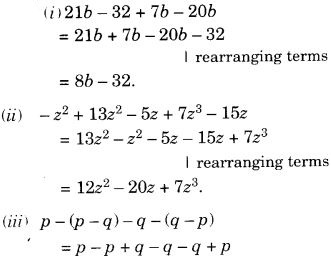Question 2.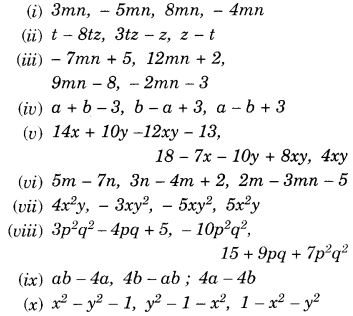Solution: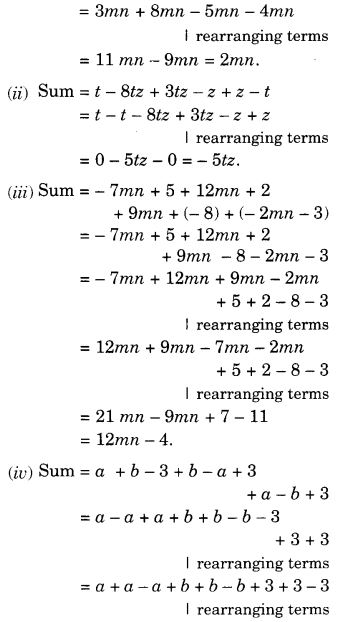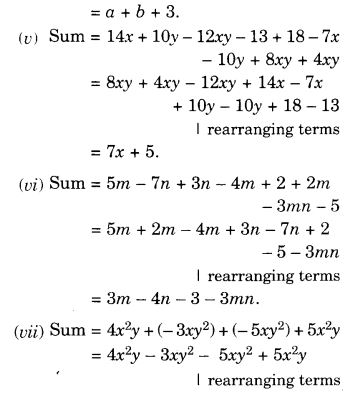Question 3.
Subtract:

1. -5y2 from y2
2. 6xy from – 12xy
3. (a – b) from (a + b)
4. a (b – 5) from b (5 – a)
5. -m2 + 5mn from 4m2 – 3mn + 8
6. -x2 + 10x – 5 from 5x – 10
7. 5a2 – 7ab + 5b2 from 3ab – 2a2 – 2b2
8. 4pq – 5q2 – 3p2 from 5p2 + 3q2 – pq.

Solution: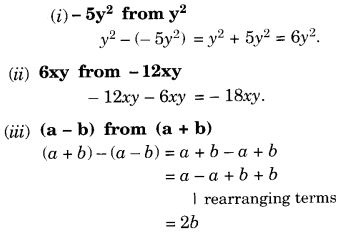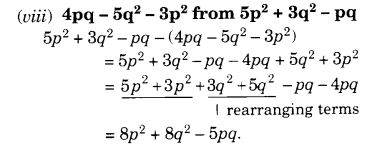Question 4.
(a) What should be added to x2 + xy + y2 to obtain 2x2 + 3xy?
(b) What should be subtracted from 2a + 8b + 10 to get -3a + 76 + 16?
Solution: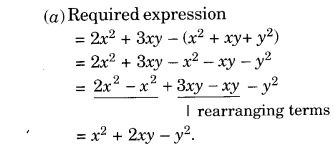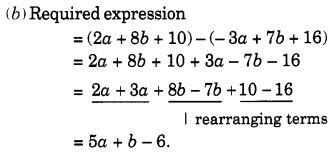Question 5.
What should be taken away from 3x2 – 4y2 + 5xy + 20 to obtain -x2 – y2 + 6xy + 20?
Solution:Question 6.
(a) From the sum of 3x – y + 11 and – y – 11, subtract 3x – y – 11.
(b) From the sum of 4 + 3x and 5 – 4x + 2x2, subtract the sum of 3x2 – 5x and -x2 + 2x + 5.
Solution: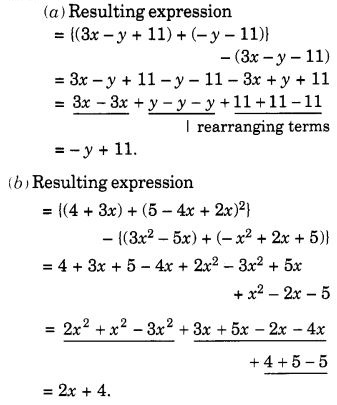We hope the NCERT Solutions for Class 7 Maths Chapter 12 Algebraic Expressions Ex 12.2 help you. If you have any query regarding NCERT Solutions for Class 7 Maths Chapter 12 Algebraic Expressions Ex 12.2, drop a comment below and we will get back to you at the earliest.